Newton's Inverse-Square Force Law Next: The Big Bang Up: Some Consequences Previous: Gravitational Collapse

### Newton's Inverse-Square Force Law

A basic test of general relativity is to check that it reduces to good old Newtonian gravity in the limit where gravitational effects are weak and velocities are small compared to the speed of light. To do this, we can use our formulation of Einstein's equation to derive Newton's inverse-square force law for a planet with mass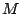and radius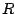. Since we can only do this when gravitational effects are weak, we must assume that the planet's radius is much greater than its Schwarzschild radius:in units where. Then the curvature of space - as opposed to spacetime - is small. To keep things simple, we make a couple of additional assumptions: the planet has uniform density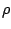, and the pressure is negligible.

We want to derive the familiar Newtonian resultgiving the radial gravitational acceleration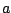of a test particle at distance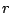from the planet's center, with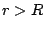of course.

To do this, let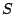be a sphere of radiuscentered on the planet. Fill the interior of the sphere with test particles, all of which are initially at rest relative to the planet. At first, this might seem like an illegal thing to do: we know that notions like `at rest' only make sense in an infinitesimal neighborhood, andis not infinitesimal. But because space is nearly flat for weak gravitational fields, we can get away with this.

We can't apply our formulation of Einstein's equation directly to, but we can apply it to any infinitesimal sphere within. In the picture below, the solid black circle represents the planet, and the dashed circle is. The interior ofhas been divided up into many tiny spheres filled with test particles. Green spheres are initially inside the planet, and red spheres are outside.Suppose we pick a tiny green sphere that lies within the planet's volume, a distance less thanfrom the center. Our formulation of Einstein's equation (2) tells us that the fractional change in volume of this sphere will be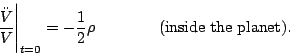On the other hand, as we saw in our discussion of tidal effects, spheres outside the planet's volume will be distorted in shape by tidal effects, but remain unchanged in volume. So, any little red sphere that lies outside the planet will undergo no change in volume at all:Thus, after a short timehas elapsed, the test particles will be distributed like this: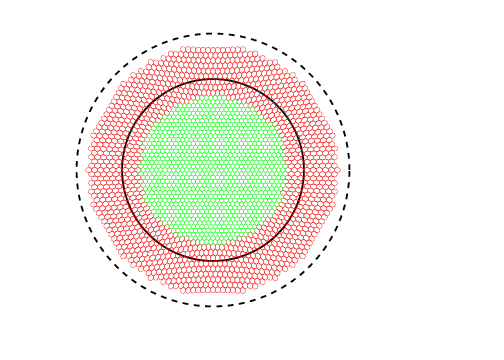Because the volume occupied by the spheres of particles within the planet went down, the whole big sphere of test particles had to shrink. Now consider the following question: what is the change in volume of the large sphere of test particles? All the little green spheres shrank by the same fractional amount: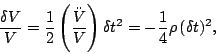to leading order in, while all the little red spheres underwent no change in volume. So, if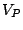stands for the volume occupied by the test particles that were initially inside the planet, andstands for the volume of the big sphere, then the overall change in volume is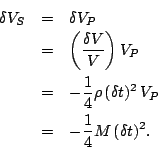We can also express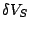in terms of the change in the sphere's radius,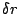: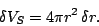Setting the two expressions forequal, we see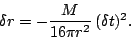But the change in radiusis just the radial displacement of a test particle on the outer edge of the sphere, so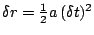whereis the radial acceleration of this particle. Plugging this into the above equation, we obtain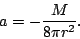We've been working all along in units in which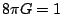. Switching back to conventional units, we obtainjust as Newton said.

Next: The Big Bang Up: Some Consequences Previous: Gravitational Collapse

© 2006 John Baez and Emory Bunn